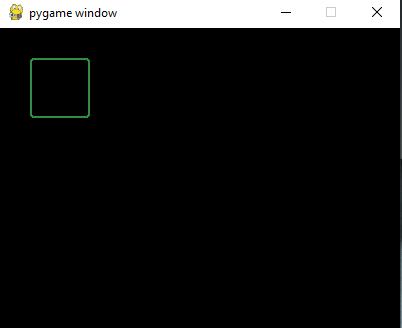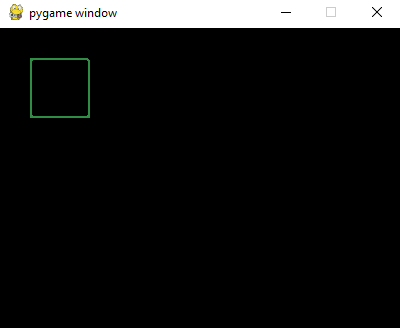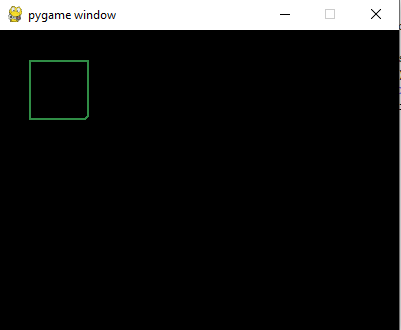Open in App
Not now

# How to draw a rectangle with rounded corner in PyGame?

• Difficulty Level : Easy
• Last Updated : 16 Mar, 2022

Pygame is a Python library designed to develop video games. Pygame adds functionality on top of the excellent SDL library. This allows you to create fully featured games and multimedia programs in the python language. In this article, we will see how can we draw a rectangle with rounded corners in Pygame.

### Functions Used:

• pygame.display.set_mode(): This function is used to initialize a surface for display. This function takes the size of the display as a parameter.
• pygame.display.flip(): This function is used to update the content of the entire display surface of the screen.
• pygame.draw.rect(): This function is used to draw a rectangle. It takes surface, color, and pygame Rect objects as input parameters and draws a rectangle on the surface.

Syntax:

Approach

• Import module
• Initialize Pygame
• Draw a rectangle with rounded borders
• Display shape

Example 1: This example draws a rectangle with all corner rounded

## Python3

 `# Importing the library``import` `pygame` `# Initializing Pygame``pygame.init()` `# Initializing surface``surface ``=` `pygame.display.set_mode((``400``, ``300``))` `# Initializing Color``color ``=` `(``48``, ``141``, ``70``)` `# Drawing Rectangle``pygame.draw.rect(surface, color, pygame.Rect(``30``, ``30``, ``60``, ``60``),  ``2``, ``3``)``pygame.display.flip()`

Output:Not just this, Pygame can be used to round even only one corner as per requirement. Given below is the implementation using the above approach.

Example 2: This example draws a rectangle with only the top right corner rounded.

## Python3

 `# Importing the library``import` `pygame` `# Initializing Pygame``pygame.init()` `# Initializing surface``surface ``=` `pygame.display.set_mode((``400``, ``300``))` `# Initializing Color``color ``=` `(``48``, ``141``, ``70``)` `# Drawing Rectangle``pygame.draw.rect(surface, color, pygame.Rect(``30``, ``30``, ``60``, ``60``),  ``2``, ``0``, ``0``, ``3``)` `# Displaying Object``pygame.display.flip()`

Output:Example 3: This example uses keyword arguments to draw a rectangle with the bottom right corner rounded.

## Python3

 `# Importing the library``import` `pygame` `# Initializing Pygame``pygame.init()` `# Initializing surface``surface ``=` `pygame.display.set_mode((``400``, ``300``))` `# Initializing Color``color ``=` `(``48``, ``141``, ``70``)` `# Drawing Rectangle``pygame.draw.rect(surface, color, pygame.Rect(``    ``30``, ``30``, ``60``, ``60``),  ``2``,  border_bottom_right_radius``=``5``)` `# Displaying Object``pygame.display.flip()`

Output:My Personal Notes arrow_drop_up